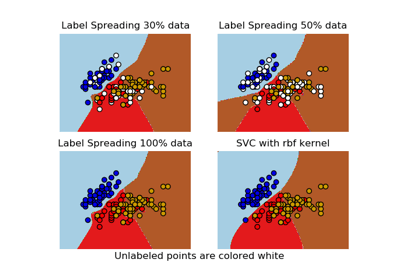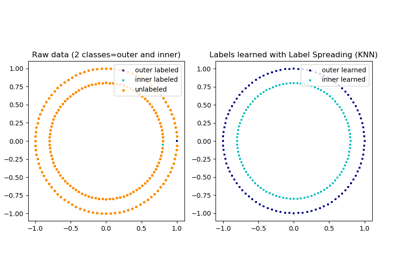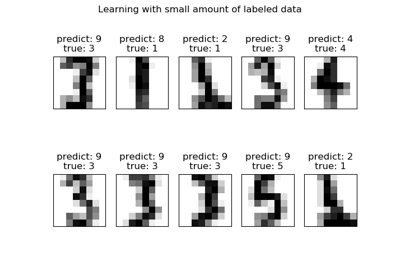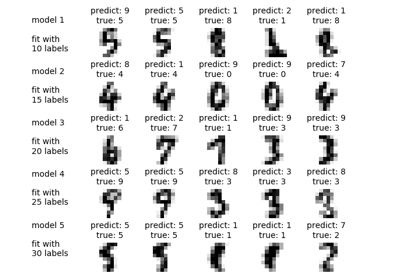# W3cubDocs

/scikit-learn

`class sklearn.semi_supervised.LabelSpreading(kernel=’rbf’, gamma=20, n_neighbors=7, alpha=0.2, max_iter=30, tol=0.001, n_jobs=None)` [source]

This model is similar to the basic Label Propagation algorithm, but uses affinity matrix based on the normalized graph Laplacian and soft clamping across the labels.

Read more in the User Guide.

Parameters: `kernel : {‘knn’, ‘rbf’, callable}` String identifier for kernel function to use or the kernel function itself. Only ‘rbf’ and ‘knn’ strings are valid inputs. The function passed should take two inputs, each of shape [n_samples, n_features], and return a [n_samples, n_samples] shaped weight matrix `gamma : float` parameter for rbf kernel `n_neighbors : integer > 0` parameter for knn kernel `alpha : float` Clamping factor. A value in [0, 1] that specifies the relative amount that an instance should adopt the information from its neighbors as opposed to its initial label. alpha=0 means keeping the initial label information; alpha=1 means replacing all initial information. `max_iter : integer` maximum number of iterations allowed `tol : float` Convergence tolerance: threshold to consider the system at steady state `n_jobs : int or None, optional (default=None)` The number of parallel jobs to run. `None` means 1 unless in a `joblib.parallel_backend` context. `-1` means using all processors. See Glossary for more details. `X_ : array, shape = [n_samples, n_features]` Input array. `classes_ : array, shape = [n_classes]` The distinct labels used in classifying instances. `label_distributions_ : array, shape = [n_samples, n_classes]` Categorical distribution for each item. `transduction_ : array, shape = [n_samples]` Label assigned to each item via the transduction. `n_iter_ : int` Number of iterations run.

`LabelPropagation`
Unregularized graph based semi-supervised learning

#### References

Dengyong Zhou, Olivier Bousquet, Thomas Navin Lal, Jason Weston, Bernhard Schoelkopf. Learning with local and global consistency (2004) http://citeseer.ist.psu.edu/viewdoc/summary?doi=10.1.1.115.3219

#### Examples

```>>> import numpy as np
>>> from sklearn import datasets
>>> rng = np.random.RandomState(42)
>>> random_unlabeled_points = rng.rand(len(iris.target)) < 0.3
>>> labels = np.copy(iris.target)
>>> labels[random_unlabeled_points] = -1
>>> label_prop_model.fit(iris.data, labels)
...
```

#### Methods

 `fit`(X, y) Fit a semi-supervised label propagation model based `get_params`([deep]) Get parameters for this estimator. `predict`(X) Performs inductive inference across the model. `predict_proba`(X) Predict probability for each possible outcome. `score`(X, y[, sample_weight]) Returns the mean accuracy on the given test data and labels. `set_params`(**params) Set the parameters of this estimator.
`__init__(kernel=’rbf’, gamma=20, n_neighbors=7, alpha=0.2, max_iter=30, tol=0.001, n_jobs=None)` [source]
`fit(X, y)` [source]

Fit a semi-supervised label propagation model based

All the input data is provided matrix X (labeled and unlabeled) and corresponding label matrix y with a dedicated marker value for unlabeled samples.

Parameters: `X : array-like, shape = [n_samples, n_features]` A {n_samples by n_samples} size matrix will be created from this `y : array_like, shape = [n_samples]` n_labeled_samples (unlabeled points are marked as -1) All unlabeled samples will be transductively assigned labels `self : returns an instance of self.`
`get_params(deep=True)` [source]

Get parameters for this estimator.

Parameters: `deep : boolean, optional` If True, will return the parameters for this estimator and contained subobjects that are estimators. `params : mapping of string to any` Parameter names mapped to their values.
`predict(X)` [source]

Performs inductive inference across the model.

Parameters: `X : array_like, shape = [n_samples, n_features]` `y : array_like, shape = [n_samples]` Predictions for input data
`predict_proba(X)` [source]

Predict probability for each possible outcome.

Compute the probability estimates for each single sample in X and each possible outcome seen during training (categorical distribution).

Parameters: `X : array_like, shape = [n_samples, n_features]` `probabilities : array, shape = [n_samples, n_classes]` Normalized probability distributions across class labels
`score(X, y, sample_weight=None)` [source]

Returns the mean accuracy on the given test data and labels.

In multi-label classification, this is the subset accuracy which is a harsh metric since you require for each sample that each label set be correctly predicted.

Parameters: `X : array-like, shape = (n_samples, n_features)` Test samples. `y : array-like, shape = (n_samples) or (n_samples, n_outputs)` True labels for X. `sample_weight : array-like, shape = [n_samples], optional` Sample weights. `score : float` Mean accuracy of self.predict(X) wrt. y.
`set_params(**params)` [source]

Set the parameters of this estimator.

The method works on simple estimators as well as on nested objects (such as pipelines). The latter have parameters of the form `<component>__<parameter>` so that it’s possible to update each component of a nested object.

Returns: self

## Examples using `sklearn.semi_supervised.LabelSpreading`Decision boundary of label propagation versus SVM on the Iris datasetLabel Propagation learning a complex structureLabel Propagation digits: Demonstrating performanceLabel Propagation digits active learning